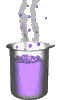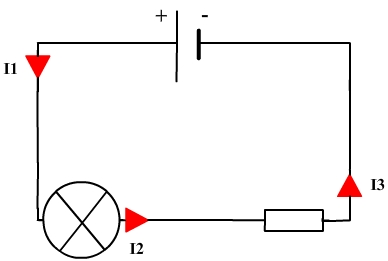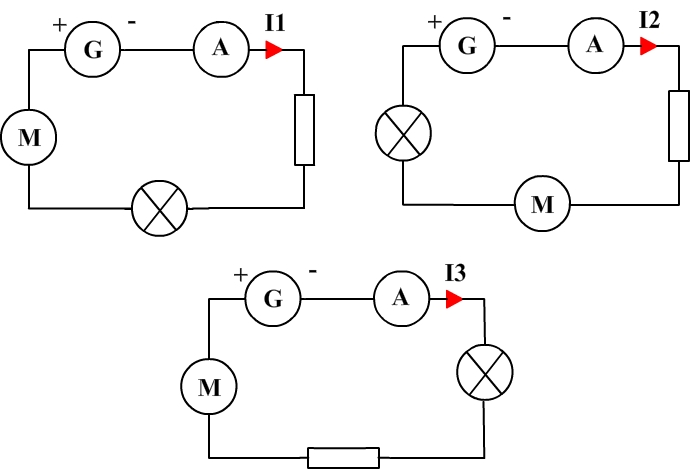Science class online
Chemistry and physics lessons

# Electricity

Free online electricity lessons for elementary school,  middle school and high school.Science class Chemistry Electricity Optics Mechanics Electricity lessons Electrical components -Two-terminal electronic components - Basic electrical components - Diodes - What's a resistor ? - How to determine a resistor value ? - Resistors effects in circuits - How to use a resistor ? - Characteristic curve of a resistor How to build simple circuits and draw diagrams - How to build a basic electrical circuit - How to draw diagrams of electric circuits The electric current - Conductors and insulators - Direction of electric current in a circuit - The dangers of electricity - Current intensity - units how to measure current ? The Voltage - The voltage and its units - How to measure a voltage ? - Voltage in open and closed circuits - Rated current and voltage for a lamp Alternating voltage and current - Alternating currents effects on led - What are alternating current and voltage ? - Periodic alternating voltage and its properties - Oscilloscope - Oscillogram - Frequency - Measuring RMS voltage with a voltmeter Serie circuits - What's a serie circuit ? - Series circuit properties - Short circuit in  series circuit - Current law in series - circuits - Voltage law in series circuits Parallel circuits - What's a parallel circuit ? - Some parallel circuits properties - Short circuits in parallel circuits - Nodes and branches in parallel circuits - Current laws in parallel circuits - Voltage laws in parallel circuits Laws of electricity - Ohm's law - Current laws in series circuits - Voltage laws in series circuits - Current laws in parallel circuits - Voltage laws in parallel circuits Generating electricity - Voltage for coil wires What is an alternator ? - Alternating currents and voltages - Generating electricity in power plants Electric power and energy - Electric power and power rating - Electric power consumption by an electrical device - Relationship between - Electric power and energy

 _____________________________________________________   _____________________________________________________

## Laws of electricity

### Current laws in series circuits

 1) The electric current in a series circuit In a series circuit the current remains unchanged all along the circuit: all electric components receive the same current. Example: In the following circuit, the electric current leaves the positive terminal, flows through the bulb, flows through the resistor and then reches the negative terminalAll along its path the current remain the same thus we can write: I1 = I2 = 3 2) Current and number of passive components in a circuit In series circuit electric, current decreases if a passive component is added: The more passive components a series circuit includes, the more current decrease. Inversely, if a passive component is taken off from a series circuit then electric current increases. ______________________________________ ______________________________________ 3) Current and order of connection Whatever is the order of connection in a series circuit, the current doesn't change. Example:These three circuits all include an electric generator, a motor, a bulb, a resistor, an ammeter and five connection wires. The only difference between these circuits is the way components are connected. We can therefore deduce that the current is the same in all circuit: I1 = I2 = I3 ______________________________________ ______________________________________
____________________________________

____________________________________